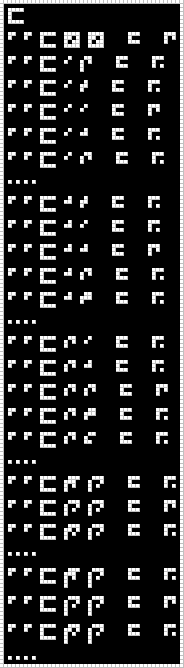# #11. Equality and Booleans¶

Note

Following documentation is a cooperative result combined from our Discord chat and numerous pull requests. Thanks to everyone who helped!

## Image¶

This image was produced from the eleventh radio transmission using previously contributed code.This partly annotated version of the image was made using code from message #3.## Interpretation¶

Operator 448 is consistent with checking whether the first number equal than the second number. We will denote it as eq.

Operator 2 is consistent with true and operator 8 is consistent with false. We will denote it as t and f respectively.

## Decoded¶

eq
ap ap eq x0 x0   =   t
ap ap eq 0 -2   =   f
ap ap eq 0 -1   =   f
ap ap eq 0 0   =   t
ap ap eq 0 1   =   f
ap ap eq 0 2   =   f
...
ap ap eq 1 -1   =   f
ap ap eq 1 0   =   f
ap ap eq 1 1   =   t
ap ap eq 1 2   =   f
ap ap eq 1 3   =   f
...
ap ap eq 2 0   =   f
ap ap eq 2 1   =   f
ap ap eq 2 2   =   t
ap ap eq 2 3   =   f
ap ap eq 2 4   =   f
...
ap ap eq 19 20   =   f
ap ap eq 20 20   =   t
ap ap eq 21 20   =   f
...
ap ap eq -19 -20   =   f
ap ap eq -20 -20   =   t
ap ap eq -21 -20   =   f
...


## Code¶

The Haskell code has been revised to decode new glyphs.

Example output: